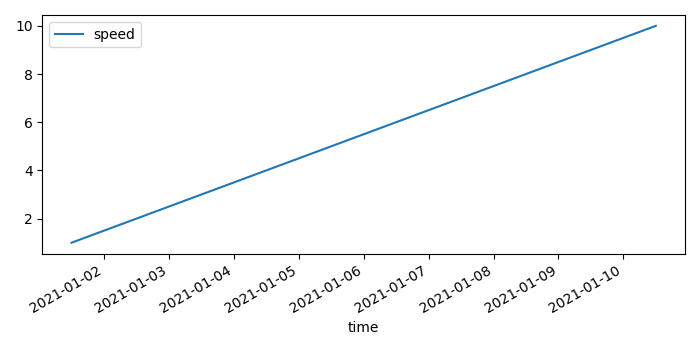# How to plot a time as an index value in a Pandas dataframe in Matplotlib?

To plot a time as an index value in a Pandas dataframe in matplotlib, we can take the following steps

## Steps

• Set the figure size and adjust the padding between and around the subplots.

• Create a Pandas dataframe with two columns, time and speed.

• Set the DataFrame index using existing columns.

• To display the figure, use Show() method.

## Example

from matplotlib import pyplot as plt
import pandas as pd
import numpy as np

# Set the figure size
plt.rcParams["figure.figsize"] = [7.00, 3.50]
plt.rcParams["figure.autolayout"] = True

# Pandas dataframe
df = pd.DataFrame(dict(time=list(pd.date_range("2021-01-01 12:00:00", periods=10)), speed=np.linspace(1, 10, 10)))

# Set the dataframe index
df.set_index('time').plot()

# Display the plot
plt.show()

## Output

It will produce the following output −